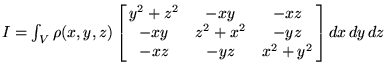# Moment of Inertia tensor SETUP, not to difficult but cant figure it out

[SOLVED] Moment of Inertia tensor SETUP, not to difficult but cant figure it out

## Homework Statement

a) For a cylinder of mass M, radius R and height h, calculate the inertia tensor about the center of mass. What are the principal axes?

## Homework Equations## The Attempt at a Solution

I need help with setting up the integral. I placed the origin at the center of the cylinder. So and i set my boundaries as = z going from -h/2 to h/2, y going from -R to R and x going from -sqrt(R^2-y^2) to sqrt(R^2-y^2).

However when i integrate Ixx I get zero and I know I am not suppose to. Is there a better choice for my boundaries?? My x boundaries are the problem here because they give me a ugly answer.

Thanks

## Answers and Replies

Try to use cylindrical coordinates, this will simplify the problem.

so for Ixx will the integral be
r^2sin^2(theta) + h^2 r^2sin(theta)dr d(theta) dz??

thank you

Last edited:
Not shperical coordinates, cylindrical ones!$$x=r\,\cos\phi,\,y=r\,\sin\phi \Rightarrow d\,x\,d\,y\,d\,z=r\,d\,r\,d\,\phi\,d\,z$$

thus $I_{xx}$ reads

$$I_{xx}=\int_V \rho(r,\phi,z)\,\left(r^2\,\sin^2\phi+z^2\right)\,r\,d\,r\,d\,\phi\,d\,z$$

Last edited:
got it!! I feel so dumb spending so much time trying to figure it out in cartesian coord. Thank you so much!!